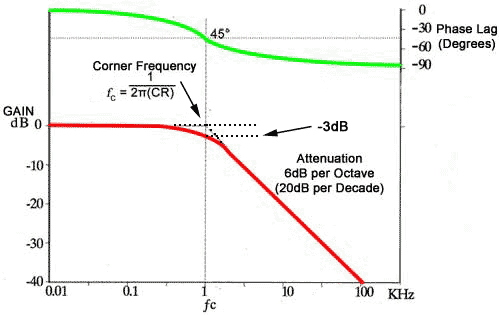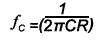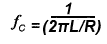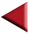# Bode Plots

• After studying this section, you should be able to describe:
• Bode Plots.
• The use of Bode plots to describe:
•   • Attenuation.
•   • Phase Change

## Showing Phase Shift and Attenuation

When considering the operation of filters, the two most important characteristics are:

• • The FREQUENCY RESPONSE, which illustrates those frequencies that will, and will not be attenuated.
• •The PHASE SHIFT created by the filter over its operating range of frequencies.

Bode Plots show both of these characteristics on a shared frequency scale making a comparison between the gain of the filter and the phase shift simple and accurate.

Frequency is plotted on the horizontal axis using a logarithmic scale, on which every equal division represents ten times the frequency scale of the previous division, this allows for a much wider range of frequency to be displayed on the graph than would be possible using a simple linear scale. Because the frequency scale increases in "Decades" (multiples of x10) it is also a convenient way to show the slope of the gain graph, which can be said to fall at 20dB per decade.

The vertical axis of the gain graph is marked off in equal divisions, but uses a logarithmic unit, the decibel (dB) to show the gain, which with simple passive filters is always unity, or a gain ratio of 1 or less, (a gain of 1 corresponds to 0dB on the logarithimic Decibel scale) . The dB units therefore have negative values indicating that the output of the filter is always less than the input, (i.e. a gain of less than 1). The upper section of the vertical axis is plotted in degrees of phase change, varying between 0 and 90° or sometimes between −90° and +90°### Fig 8.3.1 Bode Plot for a Low Pass Filter.

A typical Bode plot for a low pass filter is shown in Fig 8.3.1. Note the point called the corner frequency. This is the approximate point at which the filter becomes effective. Frequencies below this point are unaffected by the filter, while above the corner frequency, attenuation of the signal increases at a constant rate of 6dB per octave. This means that the signal output voltage is halved (−6dB) for each doubling (an octave) of the input frequency.

Alternatively the same fall off in gain may be labelled as −20dB per decade, which means that voltage gain falls by ten times (to 1/10 of its previous value) for every decade (tenfold) increase in frequency. The fall off in gain of a filter is quite linear beginning from the corner frequency (also called the cut off frequency). I.e. if the voltage gain of a low pass filter is 1 at a frequency of 1kHz, it will be 0.1 at 10kHz. The linear fall off in gain is common to both high and low pass filters, it is just the direction of the fall, increasing or decreasing with frequency, that is different. This can be seen by comparing the Bode plots for a high pass filter on the How Filters Work page and the Bode plot Fig. 8.3.2 Low Pass Filter Operation at the bottom of this page.

The corner (or cut off) frequency (ƒC) is where the active part of the gain plot begins, and the gain has fallen by −3dB. The phase lag of the output signal in a low pass filter (or phase lead in a high pass filter) is at 45°, exactly half way between its two possible extremes of 0° and 90° The corner frequency may be calculated for any two values of C and R using the formula:For LR filters the formula is:Note that the corner frequency is that point where two straight lines representing the two sections of the graph either side of ƒC would intersect. The actual curve makes a smooth transition between the horizontal and sloping sections of the graph and the gain of the filter is therefore -3dB at ƒC